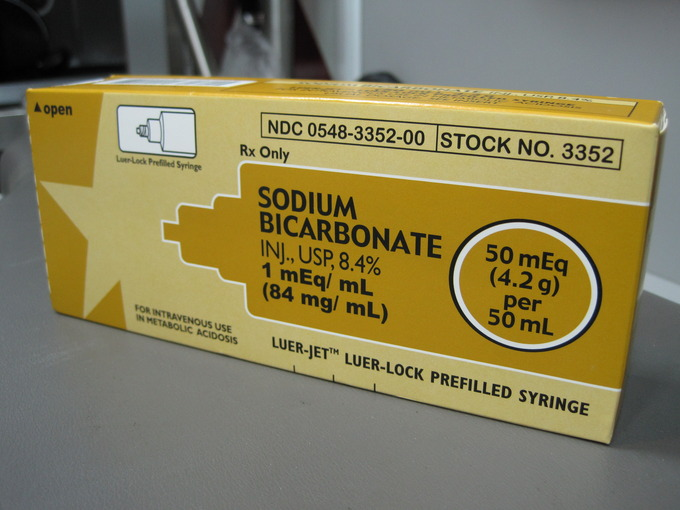## Salts that Produce Basic Solutions

#### Learning Objective

• Distinguish basic salts from non-basic salts

#### Key Points

• In acid-base chemistry, salts are ionic compounds that result from the neutralization reaction of an acid and a base.
• Basic salts contain the conjugate base of a weak acid, so when they dissolve in water, they react with water to yield a solution with pH greater than 7.0.

#### Term

• basic saltthe product of the neutralization of a strong base and a weak acid; its anion is the conjugate base of the weak acid

In acid-base chemistry, a salt is defined as the ionic compound that results from a neutralization reaction between an acid and a base. As such, salts are composed of cations (positively charged ions) and anions (negative ions), and in their unsolvated, solid forms, they are electrically neutral (without a net charge). The component ions in a salt can be inorganic; examples include chloride (Cl), the organic acetate (CH3COO), and monatomic fluoride (F), as well as polyatomic ions such as sulfate (SO42−).

## The Reaction of a Basic Salt in Water

There are several varieties of salts, and in this section we will consider basic salts. What makes a basic salt basic? It is due to the fact that the anion in the salt is the conjugate base of a weak acid. For a generalized anion B, the net ionic reaction is:

[latex]B^-(aq)+H_2O(l)\rightleftharpoons BH(aq)+OH^-(aq)[/latex]

An example of a basic salt is sodium bicarbonate, NaHCO3. The bicarbonate ion is the conjugate base of carbonic acid, a weak acid. Therefore, it reacts with water in the following fashion:

[latex]HCO_3^-(aq)+H_2O(l)\rightleftharpoons H_2CO_3(aq)+OH^-(aq)[/latex]

Because it is capable of deprotonating water and yielding a basic solution, sodium bicarbonate is a basic salt.

Other examples of basic salts include:

• Calcium carbonate (CaCO3)
• Sodium acetate (NaOOCCH3)
• Potassium cyanide (KCN)
• Sodium sulfide (Na2S)

Notice that for all of these examples, the anion is the conjugate base of a weak acid (carbonic acid, bisulfate (second dissociation step of sulfuric acid), acetic acid, hydrocyanic acid, hydrogen sulfide).

## Conjugate Bases of Weak vs. Strong Acids

Keep in mind that a salt will only be basic if it contains the conjugate base of a weak acid. Sodium chloride, for instance, contains chloride (Cl), which is the conjugate base of HCl. But because HCl is a strong acid, the Cl ion is not basic in solution, and it isn’t capable of deprotonating water.Sodium BicarbonateBecause the bicarbonate ion is the conjugate base of carbonic acid, a weak acid, sodium bicarbonate will yield a basic solution in water.